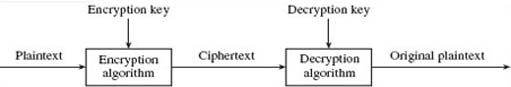Network security

Start this free course now. Just create an account and sign in. Enrol and complete the course for a free statement of participation or digital badge if available.

Free course

4 Principles of encryption

4.1 An introduction to encryption and cryptography

Section 3 has introduced you to the main threats to network security. Before I begin to examine the countermeasures to these threats I want to introduce briefly one of the fundamental building blocks of all network security. This is encryption – a process that transforms information (the plaintext) into a seemingly unintelligible form (the ciphertext) using a mathematical algorithm and some secret information (the encryption key). The process of decryption undoes this transformation using a mathematical algorithm, in conjunction with some secret value (the decryption key) that reverses the effects of the encryption algorithm. An encryption algorithm and all its possible keys, plaintexts and ciphertexts is known as a cryptosystem or cryptographic system. Figure 4 illustrates the process.Figure 4 Encryption and decryption

Cryptography is the general name given to the art and science of keeping messages secret. It is not the purpose here to examine in detail any of the mathematical algorithms that are used in the cryptographic process, but instead to provide a general overview of the process and its uses.

Modern encryption systems use mathematical algorithms that are well known and have been exposed to public testing, relying for security on the keys used. For example, a well-known and very simple algorithm is the Caesar cipher, which encrypts each letter of the alphabet by shifting it forward three places. Thus A becomes D, B becomes E, C becomes F and so on. (A cipher that uses an alphabetic shift for any number of places is also commonly referred to as a Caesar cipher, although this isn't strictly correct since the Caesar cipher is technically one in which each character is replaced by one three places to the right.) I could describe this mathematically as p + 3 = c, where p is the plaintext and c the ciphertext. For a more general equation I could write p + x = c where x could take any integer value up to 25. Selecting different values for x would obviously produce different values for c, although the basic algorithm of a forward shift is unchanged. Thus, in this example the value x is the key. (The Caesar cipher is of course too simple to be used for practical security systems.)

There are two main requirements for cryptography:

1. It should be computationally infeasible to derive the plaintext from the ciphertext without knowledge of the decryption key.
2. It should be computationally infeasible to derive the ciphertext from the plaintext without knowledge of the encryption key.

Both these conditions should be satisfied even when the encryption and decryption algorithms themselves are known.

The reason for the first condition is obvious, but probably not the second, so I shall briefly explain. In Section 3, the need to confirm authenticity was introduced. This is often also a requirement for information that is sent ‘in the clear’, that is, not encrypted. One method of authentication is for the sender and recipient to share a secret key. The sender uses the key to encrypt a copy of the message, or a portion of it, which is included with the data transfer and, on receipt, the recipient uses the key to decrypt the encrypted data. If the result matches the plaintext message, this provides a reasonable assurance that it was sent by the other key owner, and thus a check on its authenticity. (You will learn more about authentication in Section 8.) Of course, this assumes that the key has not been compromised in any way.

Modern encryption systems are derived from one of two basic systems: symmetric key (sometimes called shared key) systems, and asymmetric key (often called public key) systems.

T823_1